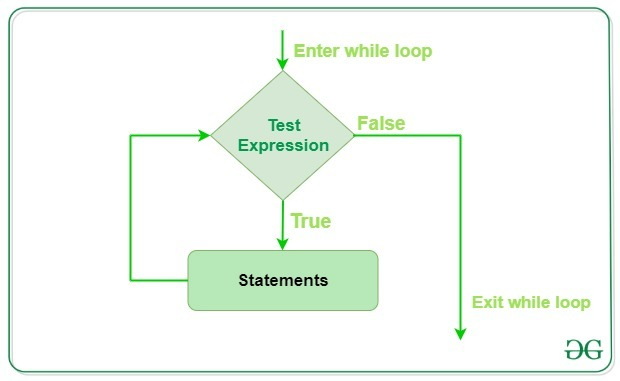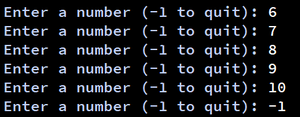# Python While Loop

## Python While Loop

Python While Loop is used to execute a block of statements repeatedly until a given condition is satisfied. And when the condition becomes false, the line immediately after the loop in the program is executed. While loop falls under the category of indefinite iteration. Indefinite iteration means that the number of times the loop is executed isn’t specified explicitly in advance.

Syntax:

```while expression:
statement(s)```

Statements represent all the statements indented by the same number of character spaces after a programming construct are considered to be part of a single block of code. Python uses indentation as its method of grouping statements. When a while loop is executed, expr is first evaluated in a Boolean context and if it is true, the loop body is executed. Then the expr is checked again, if it is still true then the body is executed again and this continues until the expression becomes false.

### Flowchart of While Loop :### Example 1: Python While Loop

 `# Python program to illustrate` `# while loop` `count ``=` `0` `while` `(count < ``3``):` `    ``count ``=` `count ``+` `1` `    ``print``(``"Hello Geek"``)`

Output

```Hello Geek
Hello Geek
Hello Geek```

In the above example, the condition for while will be True as long as the counter variable (count) is less than 3.

### Example 2: Python while loop with list

 `# checks if list still` `# contains any element` `a ``=` `[``1``, ``2``, ``3``, ``4``]` `while` `a:` `    ``print``(a.pop())`

Output

```4
3
2
1```

In the above example, we have run a while loop over a list that will run until there is an element present in the list.

## Single statement while block

Just like the if block, if the while block consists of a single statement we can declare the entire loop in a single line. If there are multiple statements in the block that makes up the loop body, they can be separated by semicolons (;).

 `# Python program to illustrate` `# Single statement while block` `count ``=` `0` `while` `(count < ``5``): count ``+``=` `1``; ``print``(``"Hello Geek"``)`

Output:

```Hello Geek
Hello Geek
Hello Geek
Hello Geek
Hello Geek```

## Loop Control Statements

Loop control statements change execution from its normal sequence. When execution leaves a scope, all automatic objects that were created in that scope are destroyed. Python supports the following control statements.

### Continue Statement

Python Continue Statement returns the control to the beginning of the loop.

### Example: Python while loop with continue statement

 `# Prints all letters except 'e' and 's'` `i ``=` `0` `a ``=` `'geeksforgeeks'` `while` `i < ``len``(a):` `    ``if` `a[i] ``=``=` `'e'` `or` `a[i] ``=``=` `'s'``:` `        ``i ``+``=` `1` `        ``continue` `        ` `    ``print``(``'Current Letter :'``, a[i])` `    ``i ``+``=` `1`

Output:

```Current Letter : g
Current Letter : k
Current Letter : f
Current Letter : o
Current Letter : r
Current Letter : g
Current Letter : k```

### Break Statement

Python Break Statement brings control out of the loop.

### Example: Python while loop with break statement

 `# break the loop as soon it sees 'e'` `# or 's'` `i ``=` `0` `a ``=` `'geeksforgeeks'` `while` `i < ``len``(a):` `    ``if` `a[i] ``=``=` `'e'` `or` `a[i] ``=``=` `'s'``:` `        ``i ``+``=` `1` `        ``break` `        ` `    ``print``(``'Current Letter :'``, a[i])` `    ``i ``+``=` `1`

Output:

`Current Letter : g`

### Pass Statement

The Python pass statement to write empty loops. Pass is also used for empty control statements, functions, and classes.

### Example: Python while loop with pass statement

 `# An empty loop` `a ``=` `'geeksforgeeks'` `i ``=` `0` `while` `i < ``len``(a):` `    ``i ``+``=` `1` `    ``pass` `  ` `print``(``'Value of i :'``, i)`

Output:

`Value of i : 13`

## While loop with else

As discussed above, while loop executes the block until a condition is satisfied. When the condition becomes false, the statement immediately after the loop is executed. The else clause is only executed when your while condition becomes false. If you break out of the loop, or if an exception is raised, it won’t be executed.

Note: The else block just after for/while is executed only when the loop is NOT terminated by a break statement.

 `# Python program to demonstrate` `# while-else loop` `i ``=` `0` `while` `i < ``4``:` `    ``i ``+``=` `1` `    ``print``(i)` `else``:  ``# Executed because no break in for` `    ``print``(``"No Break\n"``)` `i ``=` `0` `while` `i < ``4``:` `    ``i ``+``=` `1` `    ``print``(i)` `    ``break` `else``:  ``# Not executed as there is a break` `    ``print``(``"No Break"``)`

Output:

```1
2
3
4
No Break

1```

## Sentinel Controlled Statement

In this, we don’t use any counter variable because we don’t know that how many times the loop will execute. Here user decides that how many times he wants to execute the loop. For this, we use a sentinel value. A sentinel value is a value that is used to terminate a loop whenever a user enters it, generally, the sentinel value is -1.

### Example: Python while loop with user input

 `a ``=` `int``(``input``(``'Enter a number (-1 to quit): '``))` `while` `a !``=` `-``1``:` `    ``a ``=` `int``(``input``(``'Enter a number (-1 to quit): '``))`

Output:Explanation:

• First, it asks the user to input a number. if the user enters -1 then the loop will not execute
• User enter 6 and the body of the loop executes and again ask for input
• Here user can input many times until he enters -1 to stop the loop
• User can decide how many times he wants to enter input

Last Updated on October 28, 2021 by admin

## Python – How to get the last element of listPython – How to get the last element of list

Python | How to get the last element of list List, being an essential python

## Creating Pandas DataFrame from ListsCreating Pandas DataFrame from Lists

In this article, we will explore how to create a Pandas DataFrame from lists in

## Python – grid() method in TkinterPython – grid() method in Tkinter

Python | grid() method in Tkinter The Grid geometry manager puts the widgets in a 2-dimensional table.

## Print lists in Python (4 Different Ways)Print lists in Python (4 Different Ways)

Print lists in Python (All Different Ways) Using for loop : Traverse from 0 to

## Creating a Pandas SeriesCreating a Pandas Series

Pandas Series is a one-dimensional labelled array capable of holding data of any type (integer,

## median() function in Python statistics modulemedian() function in Python statistics module

median() function in Python statistics module Python is a very popular language when it comes

## Add a key value pair to dictionary in PythonAdd a key value pair to dictionary in Python

Dictionary in Python is an unordered collection of data values, used to store data values

## matplotlib.pyplot.imshow() in Pythonmatplotlib.pyplot.imshow() in Python

matplotlib.pyplot.imshow() in Python Matplotlib is a library in Python and it is numerical – mathematical extension